Fundamentals of Light & Optics

Properties of Light - Diffraction

Diffraction is the resultant of the wave-nature of light. When light waves (or any other wave) crosses the edges of obstacles, this causes the waves to bend around the edge, also if the waves pass through an aperture, this causes the light waves to spread out. The effects of diffraction are also seen if one looks closely at the boundary of shadows cast by objects, we see it is not sharp.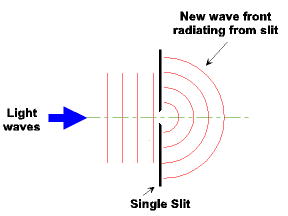Fig 2.9 - Diffraction of Light Waves

Fig 2.9 shows the principle of light diffraction. As the wavefront travels through the slit, a new spherical wavefront radiates from the slit, this is shown by the experiment conducted by Christiaan Huygens.

Huygens' Principle

In 1690, Huygens' Principle described the basis of wave propagation and the understanding of diffraction. Huygens' Principle states that: The propagation of a wave can be predicted, as each point on a propagating (advancing) wavefront is in fact an emitter of secondary wavelets which spread out spherically in all directions. The envelope of the secondary wavelets, after a small interval of time becomes the new wavefront.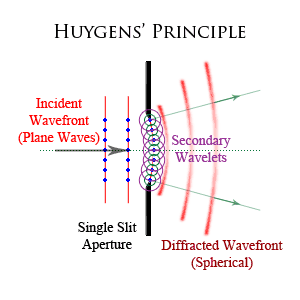Fig 2.10 - Huygens' Principle & Wavelets

Augustin-Jean Fresnel elaborated on Huygens' Principle by stating that, the amplitude of the primary wavefront at any point is the sum of the superposition of the amplitudes of the secondary wavelets at that point.

Together, Huygens - Fresnel Principle is able to describe refraction, diffraction and interference (purly, Huygens Principle, was not able to describe interference).

Applying Huygens - Fresnel Principle, we can describle the behaviour of wavefronts incident on a slit or an aperture. Each point in the slit or aperture is a point source of secondary wavelets which travel spherically away from the aperture in all directions (diffraction). The interaction of these waves give rise to interference, which we will discuss in more detail on the next page.

Classes of Diffraction

Diffraction effects are traditionally classed into two types, either Fresnel Diffraction or Fraunhofer Diffraction.

Both classes of diffraction have considerable and complex mathematical theory to derive and explain the quantum physics involved, as such we will provide an overview these classes of diffraction to cover an understanding of the concepts.

Fresnel Diffraction relates to diffraction effects in the near-field and in the immediate proximity to the diffracting object or aperture. Fresnel diffraction is a result of the spherical wavefronts illuminating the diffracting object. To better understand this type of diffraction lets consider a circular aperture, we can divide the aperture into a series of concentric annular circles or zones known as Fresnel Zones. The distance between one zone to another is one half wavelength (λ/2).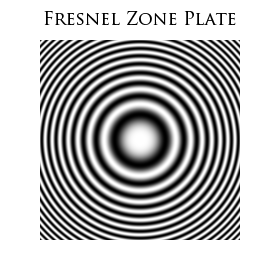Fig 2.11 - Fresnel Zone Plate

If the size (radius) of the aperture or the distance from the observing screen changes as to allow or block light from the Fresnel Zones passing through the aperture, we will observe alternating bright or dark central spot (on-axis irradiance) with concentric circular fringes off-axis. The irradiance (intensity) of the central spot oscillates between zero and 4 times that of a clear aperture if all but the first Fresnel zone are blocked. All of Huygens wavelets from the first Fresnel zone arrive in phase at the screen (observation point).

If the aperture radius or distance is changed to allow two Fresnel zones to pass, we will observe a dark central spot. As we have just covered, the difference between one zone and the next is one half of a wavelength, therefore the wavelets from the second Fresnel zone will arrive at the observation point 180° out of phase. For all odd numbered zones, waves are in phase resulting in a bright central spot, with even-numbered zones resulting in a dark central spot.

Fresnel diffraction results in a Fresnel number greater than 1. As the distance is increased, the diffracted waves become planar and are considered far-field.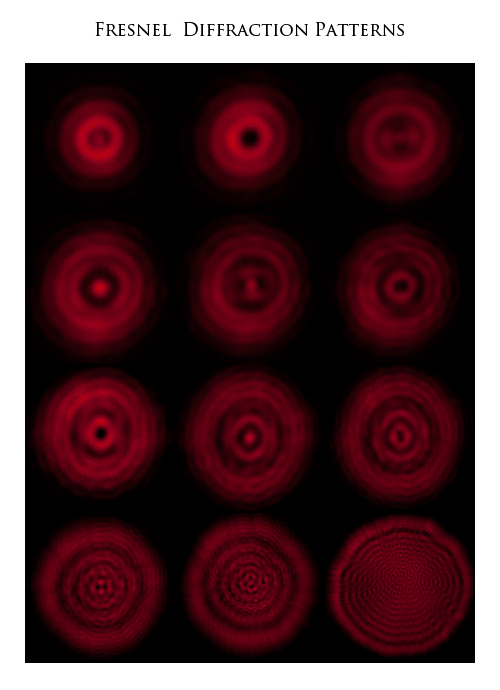Fig 2.12 - Fresnel (Near-Field) Diffraction Patterns

Fig 2.12 are actual photographs that I had taken of Fresnel diffraction. A beam from a Helium-Neon laser (632nm) is first passed through a lens then onto a circular aperture. On the opposite side of the aperture, a second lens is used to project a view of the patterns onto a viewing card. The second lens is moved in reference to the aperture. As the lens is moved away from the aperture, we are able to see the pattern change size and shape. At a point beyond the Fresnel zone, we move into the far-field, and the diffraction patterns simply change size in reference to the distance.

Fraunhofer Diffraction relates to far-field diffraction effects which result from the plane waves incident on the diffracting object. The diffraction effects are observed when Fresnel numbers are much less than 1.

The occurrence of diffraction effects are given by the Fresnel number, F;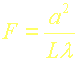Where (λ) is the wavelength, a is the characteristic size (e.g. radius) of the aperture, and L is the distance of the screen from the aperture.

Single-Slit Diffraction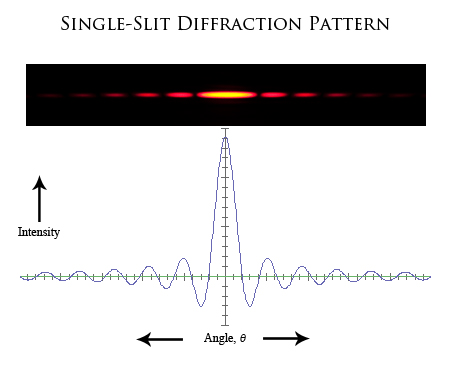Fig 2.13 - Single-Slit Diffraction

Fig 2.13 is an actual photograph that I have taken of the diffraction pattern created by passing a beam from a Helium-Neon laser with a wavelength of 632.8nm through a single-slit.

The graph below the photo represents the intensity distribution (profile) of the pattern. The central peak is the point of greatest intensity (maximum intensity) with 90.3% of the total energy distribution. As the angle increases, we see a pattern of decreasing intensities. The first intensity point either side of the maximum is known as the first minimum with 4.7% of the energy distribution, the following is the second minimum and so on.

The photo shows the first four minimums, which has only 0.5% of the total engery distribution.

Circular Aperture Diffraction

Circular apertures are important in diffraction study as they represent the typical geometry and shape of lenses, and dictates the fundamental performance limits of circular lenses.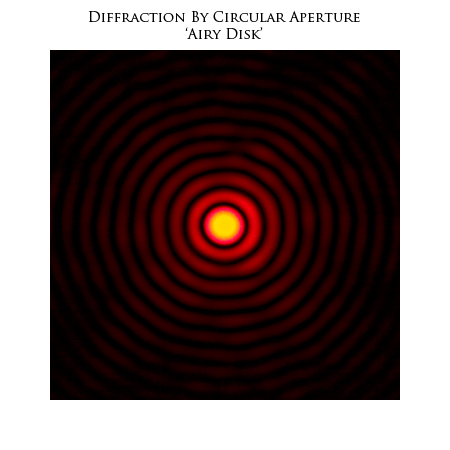Fig 2.14 - Diffraction through Circular Aperture

Fig 2.14 is an actual photo that I have taken of the resultant pattern created when a beam of light from a Helium-Neon laser at a wavelength of 632.8nm travels through a circular aperture, in this case, the aperture is a pin-hole created in foil with an ND:YAG Laser.

The central bright area is known as the Airy Disk, with the concentric rings known as the Airy pattern. The energy distribution from a circular aperture to the fourth bright ring is, 83.8% of the total energy is in the Airy Disk, following 7.2% in first bright ring, 2.8% in the second, 1.5% in the third and 1.0% in the fourth.

The diameter of the disk and patterns are governed by the wavelength and the size of the aperture. The smaller the aperture, the size of the pattern increases at a given distance and the greater the divergence of the diffracted beams of light.

The Airy disk has great importance in physics, optics and astronomy, in particular to the resolving power of imaging systems such as cameras and telescopes.

The ability for an optical system to resolve detail is limited by two main factors, the first is imperfections in the optical components, lens misalignment, aberration and other physical factors in the optical system. The second factor is a fundamental maximum ability to resolve detail of which diffraction is the limiting factor.

Light incident on a circular aperture such as a lens will exhibit diffraction, therefore by virtue of the wave-nature of light, and diffraction, light is not focused to a finite point, rather it will form an Airy disk diffraction pattern with a maximum intensity at its centre with its radius to the first null. This is known as the diffraction-limited spot-size given by:

d = 2.44 λ N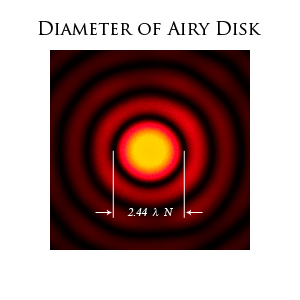Fig 2.15 - Airy Disk Diameter

Where (λ) is the wavelength of light and (N) is the f-number or focal length, (f) divided by the diameter, (D) of the optical element (lens).

An optical imaging system in which the effects of aberration no longer contribute to a decrease in resolving ability, with the only other limiting factor being that of diffraction, this is said to be a diffraction-limited system. The diffraction limit is the ultimate limit at which a system is able to resolve detail i.e. resolve the separation between two points.

When light is focused by a lens (or mirror) The resolution of an optical system is governed by the diameter of the aperture, which is inversely proportional to the wavelength of Light entering the system. The smallest detail that a diffraction-limited system is able to resolve, is given by the angle of which the first minimum occurs.

Sin θ = 1.22(λ / d)

Where (λ) is the wavelength of light and (d) the diameter of the aperture of the imaging system (or lens).

Using photography as an example of an imaging application, the resolution of the lens and image sensor (the optical system) is ultimately limited by diffraction. From a photographers point of view, resolution translates to sharpness of the image.

We know that the diameter of the aperture plays and important role in diffraction, that is, as the size of the aperture is decreased diffraction increases. Relating to photography, decreasing the aperture, i.e. stopping down the lens, will result in increase in apparent sharpness (depth-of-field), although with the advent of digital photography, this has introduced a new problem that we now have to contend with, that is the diffraction-limit.

The point has now been reached where the resolving power of the lenses and image sensors combined is much greater than the days of silver-halide film, where now the effects of diffraction are a contributing factor in how 'sharp' we can resolve an image. We are now at the ultimate (physical) limits on how much further resolving power can be achieved.

We have just learnt that a diffraction-limited system, is one is which all other causes contributing to a reduction in resolving power (i.e. sharpness), are no longer contributing factor, rather the only factor remaining is diffraction. As the effects of Diffraction increase, the resolving ability decreases to a point where we are no longer able to resolve two separate points or images.

With respect to imaging systems, the Rayleigh Criterion states that:

"The images of two different points sources are regarded as just resolved when the center of the Airy disk of one image overlaps first minimum (dark ring) in the Airy pattern of the other. If the distance is greater, the two points are well resolved and if it is smaller, they are not resolved".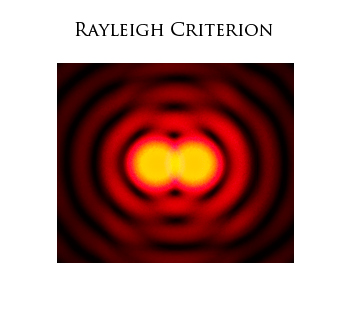Fig 2.16 - Rayleigh Criterion (Just Resolved)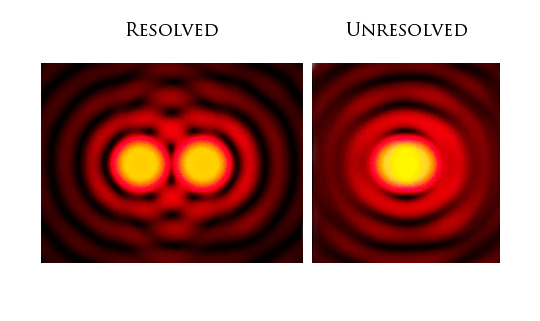Fig 2.17 - Resolved & Unresolved Points

Now that we have an understand of diffraction, this leads onto another aspect of the wave-nature of light, where diffracted wavefronts will interact with other wavefronts and set about interfering with one another.

In the next section, we will discuss the interference of light

This Document Complies to W3C XHTML 1.0 Strict Standards